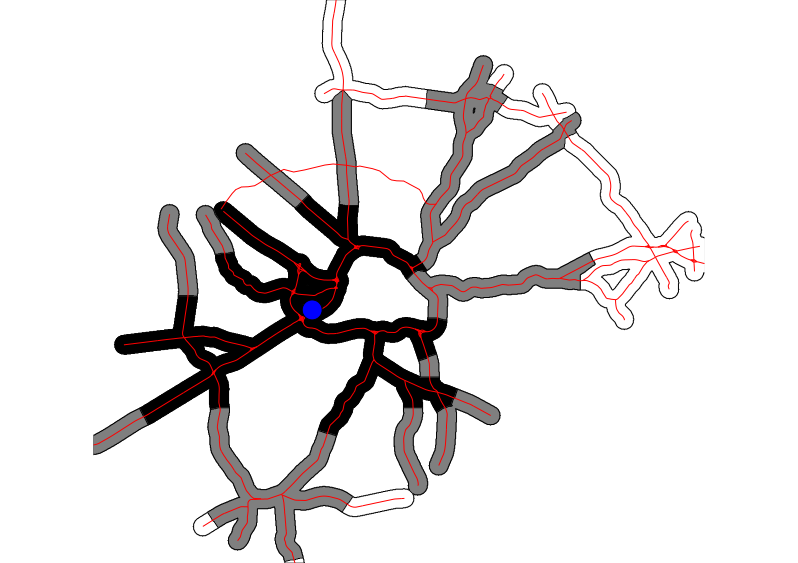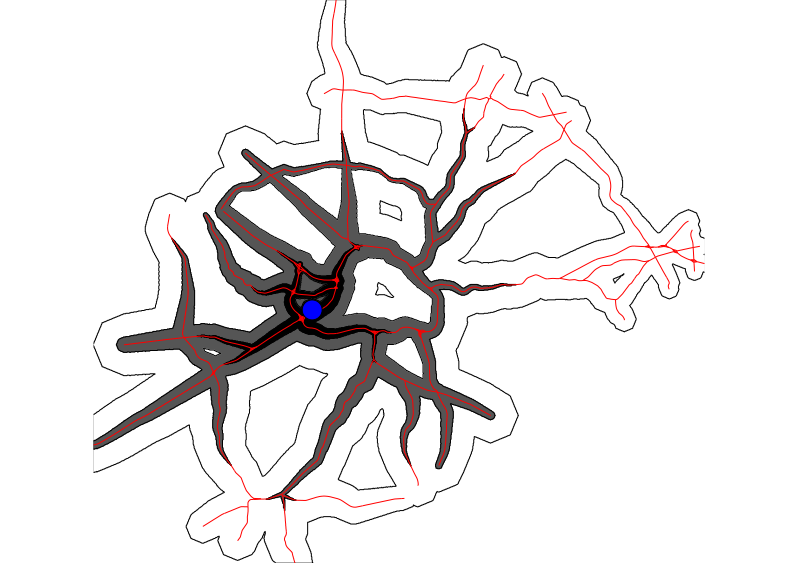## NAME

v.isochrones - Creates isochrones from a road map and starting points

## KEYWORDS

vector, network, isochrones

## SYNOPSIS

v.isochrones
v.isochrones --help
v.isochrones [-i] map=name roads_layer=string [node_layer=string] cost_column=name start_points=name isochrones=name time_steps=float[,float,...] [timemap=name] [offroad_speed=float] [memory=integer] [max_distance=float] method=string [--overwrite] [--help] [--verbose] [--quiet] [--ui]

### Flags:

-i
Create individual isochrone map for each starting point
--overwrite
Allow output files to overwrite existing files
--help
Print usage summary
--verbose
Verbose module output
--quiet
Quiet module output
--ui
Force launching GUI dialog

### Parameters:

map=name [required]
Or data source for direct OGR access
Layer number of the roads with relevant attributes
Vector features can have category values in different layers. This number determines which layer to use. When used with direct OGR access this is the layer name.
Default: 1
node_layer=string
Layer number of the nodes on the network (for method=v.net.iso)
Vector features can have category values in different layers. This number determines which layer to use. When used with direct OGR access this is the layer name.
Default: 2
cost_column=name [required]
Name of speed attribute column (in km/h) or cost column (in minutes)
start_points=name [required]
Vector map with starting points for isochrones
Or data source for direct OGR access
isochrones=name [required]
Output vector map with isochrone polygons (Output prefix with flag -i)
Name for output vector map
time_steps=float[,float,...] [required]
Time steps of isochrones (in minutes)
timemap=name
Optional output raster map with continuous time from starting points
Name for output raster map
Speed for off-road areas (in km/h > 0)
Default: 5.0
memory=integer
Amount of memory (in MB) use
Default: 300
max_distance=float
Maximum distance (m) from network to include into isochrones
method=string [required]
Method to use for isochrone calculation
Options: v.net.iso, r.cost
Default: v.net.iso

## DESCRIPTION

v.isochrones creates a vector polygon map of isochrones (isochrones) based on a roads map (map) with speed or cost attribute (cost_column), one or several starting points (start_points) and time steps (in minutes) for the isochrones (time_steps). The module is actually a front-end to different GRASS GIS modules, and the user can chose the approach with the method option (see the notes section for details).

The spatial precision of the analysis is defined by the current computational region. The user can define this with g.region. Care should be taken to not define a too high resolution for the spatial extent covered. Otherwise the user might be confronted with memory issues.

## NOTES

Two approaches are currently implemented in the module:

The first (default) approach is based on v.net.iso. The output of that module is then used to assign isochrone values to all pixels based on the nearest road segment that is within a given distance (max_distance). The -i flag allows to calculate a separate isochrone map for each starting point. For this approach, the input road map currently has to be prepared by adding all nodes (using v.net with 'operation=nodes' and the '-c' flag. For each starting point, the algorithm then finds the closest node on the network and calculates the isochrones from there. This allows to use the same network for many analyses without having to go through the time of adding the starting points at each run. In addition, a cost_column has to be present in the attribute table containing for each line segment the time of traversal in minutes (i.e. length/speed).

The second approach is based on r.cost. It transforms the roads to raster (here cost_column has to point to an attribute column containing speed in km/h), assigning a user chosen offroad_speed to all offroad pixels, calculates time cost from the starting points and then transforms the result into discrete vector polygons based on the time steps chosen. Optionally, the user can chose to keep the a raster output of r.cost (timemap). Current region settings are used to define the maximal extension and the resolution at which the cost map is calculated. The memory option allows to decide how much memory the r.cost module can use (see the r.cost man page for more details). One big advantage of the this second approach is that the road network does not have to be topologically clean in order to get meaningful results.

## EXAMPLE

```v.net -c input=roadsmajor operation=nodes output=myroads
v.db.update myroads col=speed value=50 where="speed is null"

echo "634637|224663" | v.in.ascii input=- output=start x=1 y=2

v.isochrones map=myroads cost_column=cost start_points=start isochrones=isochrones_vnetiso time_steps=15,30,60 \
max_distance=1000 method=v.net.iso
```15, 30 and 60 minute isochrones from the start point using method v.net.iso
```v.isochrones map=myroads cost_column=speed start_points=start isochrones=isochrones_rcost time_steps=15,30,60 \
method=r.cost memory=1000
```15, 30 and 60 minute isochrones from the start point using method r.cost